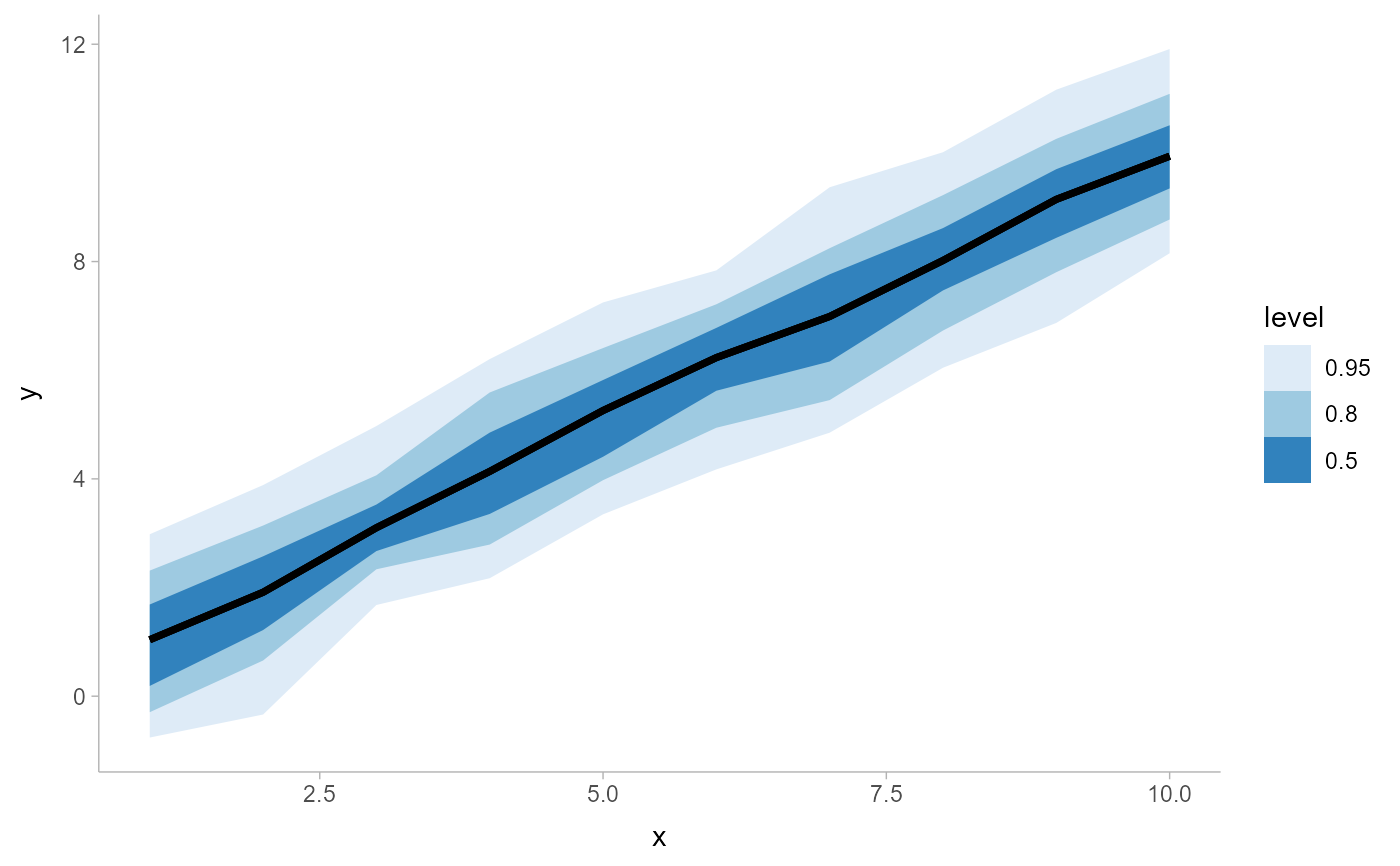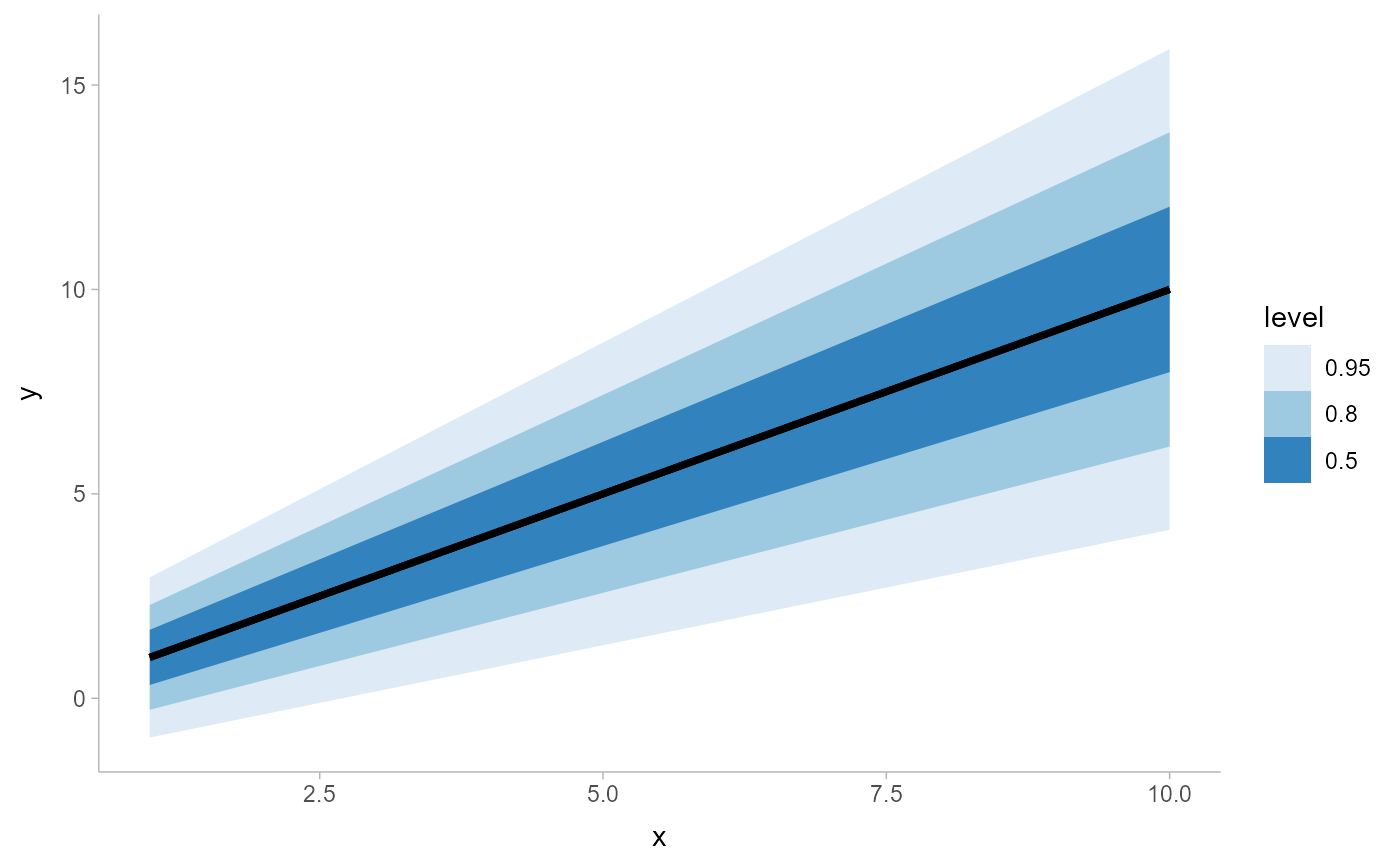A combination of stat_slabinterval() and geom_lineribbon() with sensible defaults. While geom_lineribbon is intended for use on data frames that have already been summarized using a point_interval() function, stat_lineribbon is intended for use directly on data frames of draws, and will perform the summarization using a point_interval() function; stat_dist_lineribbon is intended for use on analytical distributions through the dist, arg1, ... arg9, and args aesthetics.

stat_lineribbon(
mapping = NULL,
data = NULL,
geom = "lineribbon",
position = "identity",
...,
interval_function = NULL,
interval_args = list(),
point_interval = median_qi,
.width = c(0.5, 0.8, 0.95),
na.rm = FALSE,
show.legend = NA,
inherit.aes = TRUE,
.prob,
fun.data,
fun.args
)

stat_dist_lineribbon(
mapping = NULL,
data = NULL,
geom = "lineribbon",
position = "identity",
...,
n = 501,
.width = c(0.5, 0.8, 0.95),
na.rm = FALSE,
show.legend = NA,
inherit.aes = TRUE
)

## Arguments

mapping Set of aesthetic mappings created by aes() or aes_(). If specified and inherit.aes = TRUE (the default), it is combined with the default mapping at the top level of the plot. You must supply mapping if there is no plot mapping. The data to be displayed in this layer. There are three options: If NULL, the default, the data is inherited from the plot data as specified in the call to ggplot(). A data.frame, or other object, will override the plot data. All objects will be fortified to produce a data frame. See fortify() for which variables will be created. A function will be called with a single argument, the plot data. The return value must be a data.frame, and will be used as the layer data. A function can be created from a formula (e.g. ~ head(.x, 10)). Use to override the default connection between geom_lineribbon and stat_lineribbon. Position adjustment, either as a string, or the result of a call to a position adjustment function. Other arguments passed to layer(). They may also be arguments to the paired geom (e.g., geom_pointinterval()) Custom function for generating intervals (for most common use cases the point_interval argument will be easier to use). This function takes a data frame of aesthetics and a .width parameter (a vector of interval widths), and returns a data frame with columns .width (from the .width vector), .value (point summary) and .lower and .upper (endpoints of the intervals, given the .width). Output will be converted to the appropriate x- or y-based aesthetics depending on the value of orientation. If interval_function is NULL, point_interval is used instead. Additional arguments passed to interval_function or point_interval. A function from the point_interval() family (e.g., median_qi, mean_qi, etc). This function should take in a vector of value, and should obey the .width and .simple_names parameters of point_interval() functions, such that when given a vector with .simple_names = TRUE should return a data frame with variables .value, .lower, .upper, and .width. Output will be converted to the appropriate x- or y-based aesthetics depending on the value of orientation. See the point_interval() family of functions for more information. The .width argument passed to interval_function or point_interval. If FALSE, the default, missing values are removed with a warning. If TRUE, missing values are silently removed. Should this layer be included in the legends? NA, the default, includes if any aesthetics are mapped. FALSE never includes, and TRUE always includes. If FALSE, overrides the default aesthetics, rather than combining with them. This is most useful for helper functions that define both data and aesthetics and shouldn't inherit behaviour from the default plot specification, e.g. borders(). Deprecated. Use .width instead. Deprecated. Use point_interval or interval_function instead. Deprecated. Use interval_args instead. Number of points at which to evaluate slab_function

## Value

A ggplot2::Stat representing a combined line+uncertainty ribbon geometry which can be added to a ggplot() object.

See geom_lineribbon() for the geom version, intended for use on points and intervals that have already been summarized using a point_interval() function. See stat_pointinterval() for a similar stat intended for point summaries and intervals.

## Examples


library(dplyr)
library(ggplot2)
library(distributional)

tibble(x = 1:10) %>%
group_by_all() %>%
do(tibble(y = rnorm(100, .\$x))) %>%
ggplot(aes(x = x, y = y)) +
stat_lineribbon() +
scale_fill_brewer()tibble(
x = 1:10,
sd = seq(1, 3, length.out = 10)
) %>%
ggplot(aes(x = x, dist = dist_normal(x, sd))) +
stat_dist_lineribbon() +
scale_fill_brewer()